Next: Acceleration Up: Motion in 1 dimension Previous: Displacement

## Velocity

Both Fig. 3 and formula (11) effectively specify the location of the body whose motion we are studying as time progresses. Let us now consider how we can use this information to determine the body's instantaneous velocity as a function of time. The conventional definition of velocity is as follows:
Velocity is the rate of change of displacement with time.
This definition implies that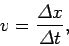(12)

whereis the body's velocity at time, and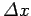is the change in displacement of the body between timesand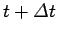.

How should we choose the time interval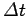appearing in Eq. (12)? Obviously, in the simple case in which the body is moving with constant velocity, we can makeas large or small as we like, and it will not affect the value of. Suppose, however, thatis constantly changing in time, as is generally the case. In this situation,must be kept sufficiently small that the body's velocity does not change appreciably between timesand. Ifis made too large then formula (12) becomes invalid.

Suppose that we require a general expression for instantaneous velocity which is valid irrespective of how rapidly or slowly the body's velocity changes in time. We can achieve this goal by taking the limit of Eq. (12) asapproaches zero. This ensures that no matter how rapidlyvaries with time, the velocity of the body is always approximately constant in the intervalto. Thus,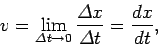(13)

where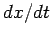represents the derivative ofwith respect to. The above definition is particularly useful if we can represent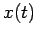as an analytic function, because it allows us to immediately evaluate the instantaneous velocity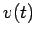via the rules of calculus. Thus, ifis given by formula (11) then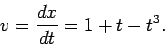(14)

Figure 4 shows the graph ofversusobtained from the above expression. Note that whenis positive the body is moving to the right (i.e.,is increasing in time). Likewise, whenis negative the body is moving to the left (i.e.,is decreasing in time). Finally, when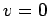the body is instantaneously at rest.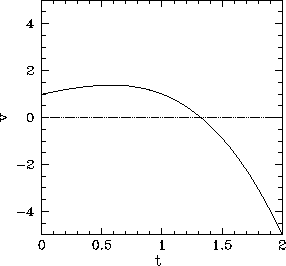The terms velocity and speed are often confused with one another. A velocity can be either positive or negative, depending on the direction of motion. The conventional definition of speed is that it is the magnitude of velocity (i.e., it iswith the sign stripped off). It follows that a body can never possess a negative speed.Next: Acceleration Up: Motion in 1 dimension Previous: Displacement
Richard Fitzpatrick 2006-02-02